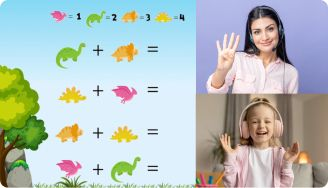# Basic Fractions Worksheets

As kids climb up the academic ladder, they begin to learn core mathematical concepts such as fractions. Although fractions can be a tough topic, there’s simply no escaping it. Kids will have to sit through this topic in order to advance to the next stage. But there is a way to ease this encounter for them by providing a guide in the form of basic fractions worksheets.

Leave your email and we will send you worksheets

worksheets sent successfully

## About Basic Fractions Worksheets for Kids

The basic fractions worksheet is designed to guide young learners in the world of fractions. It contains several exercises that cover all the basics (types of fractions, fractional operations, etc). Through constant practice and use of this worksheet, kids will develop the necessary math skills.

1:1 Math Lessons

Want to raise a genius?
Start learning Math with Brighterly## Benefits of Basic Fractions Worksheets PDF

The fractions worksheet is a handy tool for parents, tutors, and young learners. It helps students acquire an in-depth knowledge of fractions and learn how to work with them. With these tools, kids can begin to apply arithmetic skills in real-life situations.

Leave your email and we will send you worksheets

worksheets sent successfully### Basic Fractions Worksheets PDF

View worksheet

Basic Fraction Worksheets### Basic Fractions Worksheets PDF

View worksheet

Basic Fractions Worksheet### Basic Fractions Worksheets PDF

View worksheet

Basic Fractions Worksheets PDF### Basic Fractions Worksheets PDF

View worksheet

Basic Fractions Worksheets For Kids

### More Fractions Worksheets

Book 1 to 1 Math Lesson• Specify your child’s math level
• Get practice worksheets for self-paced learning
• Your teacher sets up a personalized math learning plan for your childBook 1 to 1 Math Lesson